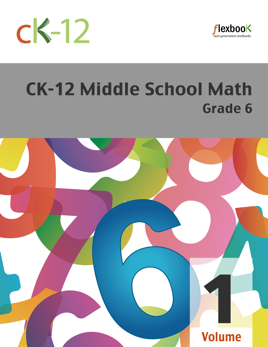## Publisher Description

CK-12's Middle School Math Grade 6 covers the fundamentals of fractions, decimals, and geometry. Also explored are units of measurement, graphing concepts, and strategies for utilizing the book’s content in practical situations.  Volume 1 includes the first 6 chapters - Number Sense and Variable Expressions, Statistics and Measurement, Addition and Subtraction of Decimals, Multiplication and Division of Decimals, Number Patterns and Fractions, and Addition and Subtraction of Fractions.